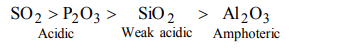## Classification of Elements and Periodicity in Properties Questions and Answers Part-8

1. Among the elements Ca, Mg, P and Cl, the order of increasing atomic radii is :
a) Ca < Mg < P < Cl
b) Mg < Ca < Cl < P
c) Cl < P < Mg < Ca
d) P < Cl < Ca < Mg

Explanation:2. What is the value of electron gain enthalpy of Na+ if IE1 of Na = 5.1 eV ?
a) –5.1 eV
b) –10.2 eV
c) +2.55 eV
d) +10.2 eV

Explanation: IE1 of Na = – Electron gain enthalpy of Na+ = – 5.1 eV

3. According to the Periodic Law of elements, the variation in properties of elements is related to their
a) nuclear masses
b) atomic numbers
c) nuclear neutron-proton number ratios
d) atomic masses

Explanation: The properties of elements change with a change in atomic number.

4. Which is the correct order of ionic sizes (At. No. : Ce = 58, Sn = 50, Yb = 70 and Lu = 71) ?
a) Ce > Sn > Yb > Lu
b) Sn > Ce > Yb > Lu
c) Lu > Yb > Sn > Ce
d) Sn > Yb > Ce > Lu

Explanation: Correct order of ionic size is Sn > Ce > Yb > Lu

5. Which one of the following ions has the highest value of ionic radius ?
a) $O^{2-}$
b) $B^{3+}$
c) $Li^{+}$
d) $F^{-}$

Explanation: The ionic radii follows the order $O^{2-}$ > $F^{-}$ > $Li^{+}$ > $B^{3+}$

6. Among $AI_{2}O_{3},SiO_{2},P_{2}O_{3} and SO_{2}$     the correct order of acid strength is
a) $AI_{2}O_{3}<SiO_{2}<SO_{2}<P_{2} O_{3}$
b) $SiO_{2}<SO_{2}<AI_{2}O_{3}<P_{2} O_{3}$
c) $SO_{2}<P_{2}O_{3}<SiO_{2}<AI_{2}O_{3}$
d) $AI_{2}O_{3}<SiO_{2}<P_{2}O_{3}<SO_{2}$

Explanation:7. Beyllium and aluminium exhibit many properties which are similar. But, the two elements differ in
a) forming covalent halides
b) forming polymeric hydrides
c) exhibiting maximum covalency in compounds
d) exhibiting amphoteric nature in their oxides

Explanation: The valency of beryllium is +2 while that of aluminium is +3

8. Which of the following oxides is amphoteric in character?
a) $SnO_{2}$
b) $SiO_{2}$
c) $CO_{2}$
d) CaO

Explanation:9. In which of the following arrangements, the order is NOT according to the property indicated against it?
a) Li < Na < K < Rb : Increasing metallic radius
b) I < Br < F < Cl : Increasing electron gain enthalpy (with negative sign)
c) B < C < N < O : Increasing first ionization enthalpy
d) $AI^{3+}<Mg^{2+}<Na^{+}<F^{-}$     : Increasing ionic size